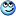# Derivative of Determinant of Metric Tensor With Respect to Entries

• yucheng

#### yucheng

Homework Statement
We are to show that $\frac{\partial Z}{\partial Z_{ij}} = Z Z^{ij}$ See https://math.stackexchange.com/questions/4141664/derivative-of-metric-tensor-with-respect-to-entries

P.S. this is actually part of the proof of the Voss-Weyl formula given in Pavel Grinfeld's book but the author does not care to derive it...
Relevant Equations
N/A
We know that the cofactor of determinant ##A##, is
$$\frac{\partial A}{\partial a^{r}_{i}} = A^{i}_{r} = \frac{1}{2 !}\delta^{ijk}_{rst} a^{s}_{j} a^{t}_{k} = \frac{1}{2 !}e^{ijk} e_{rst} a^{s}_{j} a^{t}_{k}$$

By analogy,
$$\frac{\partial Z}{\partial Z_{ij}} = \frac{1}{2 !}e^{ikl} e^{jmn} Z_{km} Z_{ln} = \frac{Z}{2 !} \epsilon^{ikl} \epsilon^{jmn} Z_{km} Z_{ln}$$?

As $$\epsilon^{ijk} = \frac{e^{ijk}}{\sqrt{Z}}$$. However, we should note that Z is **not** a tensor, since we only have relative levi-civita symbols, but if we substitute absolute levi-civita symbols, then it is indeed one (second equality)? Wait, I'm not sure whether one should call the former, ##\epsilon## 'absolute' and the latter, ##e## 'relative'...

Hmmm, this looks interesting. Would you give any hints as to what's wrong? Thanks in advance!

Last edited:

Use that ln(det(X)) = tr(ln(X)) and differentiate.

•yucheng
Use that ln(det(X)) = tr(ln(X)) and differentiate.
I see that you've given this hint somewhere else as well. Reminds me of exponentiating an operator... Oh well, I have got to learn it... Thanks!

Did I not format the homework statement properly?... $$\frac{\partial Z}{\partial Z_{ij}} = Z Z^{ij}$$

Where ##Z## is the determinant.

It's okay. Orodruin already gave you a hint:\begin{align*}
\dfrac{1}{|Z|} \dfrac{\partial |Z|}{\partial Z_{ij}} &= \dfrac{\partial \log |Z|}{\partial Z_{ij}} = \mathrm{Tr} \left( Z^{-1} \frac{\partial Z}{\partial Z_{ij}} \right)
\end{align*}Do you know how to take the trace?

•yucheng
It's okay. Orodruin already gave you a hint:\begin{align*}
\dfrac{1}{|Z|} \dfrac{\partial |Z|}{\partial Z_{ij}} &= \dfrac{\partial \log |Z|}{\partial Z_{ij}} = \mathrm{Tr} \left( Z^{-1} \frac{\partial Z}{\partial Z_{ij}} \right)
\end{align*}Do you know how to take the trace?
I am confused with traces and logs of matrices actually, but let me have a look. (I know the definition, trace is the sum of the diagonal terms, log: expand log in terms of the power series of the matrix)

It's okay. Orodruin already gave you a hint:\begin{align*}
\dfrac{1}{|Z|} \dfrac{\partial |Z|}{\partial Z_{ij}} &= \dfrac{\partial \log |Z|}{\partial Z_{ij}} = \mathrm{Tr} \left( Z^{-1} \frac{\partial Z}{\partial Z_{ij}} \right)
\end{align*}Do you know how to take the trace?
wait

\begin{align*}
\dfrac{1}{|Z|} \dfrac{\partial |Z|}{\partial Z_{ij}} = \mathrm{Tr} \left( Z^{-1} \frac{\partial Z}{\partial Z_{ij}} \right)
\end{align*}
and isn't

\begin{align*}
\dfrac{1}{|Z|} \dfrac{\partial |Z|}{\partial Z_{ij}} = \left( Z^{-1} \frac{\partial Z}{\partial Z_{ij}} \right)
\end{align*}

?

Because they are scalars!

Wait this is confusing did you intend ##|Z|## to be the determinant while ##Z## to be the metric tensor?

No. Remember that the trace of a matrix is ##\mathrm{Tr}(A) = {A^i}_i## with summation implied over repeated indices. Matrix multiplication is ##A = BC \iff {A^i}_j = {B^i}_k {C^k}_j## and the trace of this product is then ##{A^i}_i = {B^i}_k {C^k}_i##.

In the context of this step, \begin{align*}
{\left( Z^{-1} \frac{\partial Z}{\partial Z_{ij}}\right)^k}_l = Z^{km} {\frac{\partial {Z_{ml}}}{\partial Z_{ij}}}
\end{align*}What is the trace?

•yucheng
Why am I switching between matrices and tensorsWait this is confusing did you intend ##|Z|## to be the determinant while ##Z## to be the metric tensor?
Yes, I am denoting by ##Z## a matrix.

Finally, found my pen and paper.

\begin{align*}
\dfrac{1}{|Z|} \dfrac{\partial |Z|}{\partial Z_{ij}} &= \mathrm{Tr} \left( Z^{-1} \frac{\partial Z}{\partial Z_{ij}} \right)
\end{align*}

since $$Z = [Z_{ij}]$$
, $$Z^{-1} = [Z^{ij}]$$
, $$\mathrm{Tr} \left(AB\right) = A^{i}_{j} B^{j}_{i}$$

We see that
$$RHS = Z^{lm} \frac{\partial Z_{lm}}{\partial Z_{ij}}$$

and combining LHS and RHS

$$\dfrac{1}{|Z|} \dfrac{\partial |Z|}{\partial Z_{ij}}= Z^{lm} \frac{\partial Z_{lm}}{\partial Z_{ij}} = Z^{lm} \delta^{ij}_{lm} = Z^{ij}$$

In conclusion,

$$\dfrac{1}{|Z|} \dfrac{\partial |Z|}{\partial Z_{ij}}= Z^{ij}$$

or

$$\dfrac{\partial |Z|}{\partial Z_{ij}}= |Z| Z^{ij}$$

Thanks @Orodruin and @ergospherical !

•ergospherical
Nice.No. Remember that the trace of a matrix is ##\mathrm{Tr}(A) = {A^i}_i## with summation implied over repeated indices. Matrix multiplication is ##A = BC \iff {A^i}_j = {B^i}_k {C^k}_j## and the trace of this product is then ##{A^i}_i = {B^i}_k {C^k}_i##.

In the context of this step, \begin{align*}
{\left( Z^{-1} \frac{\partial Z}{\partial Z_{ij}}\right)^k}_l = Z^{km} {\frac{\partial {Z_{ml}}}{\partial Z_{ij}}}
\end{align*}What is the trace?
Just a quick question, what is $$\frac{\partial {Z}}{\partial Z_{ij}}$$? Do we take the partial derivative of each entry of the metric tensor and get back the matrix with the same dimensions?

Yeah, it's a matrix with components ##\dfrac{\partial Z_{kl}}{\partial Z_{ij}}## in the ##(k,l)^{\mathrm{th}}## position.

•yucheng